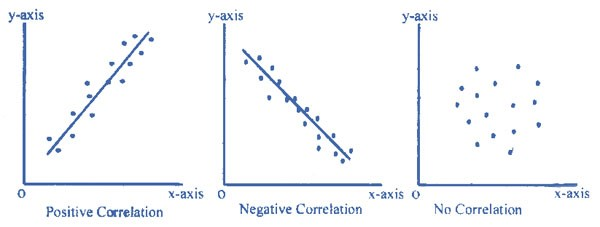# Positive and Negative Correlation

Correlation can be defined as a statistical tool that defines the relationship between two variables. For, eg: correlation may be used to define the relationship between the price of a good and its quantity demanded. It explains how two variables are related but do not explain any cause-effect relation. It only gives an understanding as to the direction and intensity of relation between two variables. Correlation can be of two types:

#### A) Positive Correlation

A correlation in the same direction is called a positive correlation. If one variable increases the other also increases and when one variable decreases the other also decreases. For example, the length of an iron bar will increase as the temperature increases.

Two variables are positively correlated when they move together in the same direction. In economics, quantity supplied increases as the price increases. This is because sellers find it profitable to sell when the prices are high, so they will sell more. Thus, we can call price and quantity supplied to be positively correlated. This is also called the law of supply.

#### B) Negative Correlation

Correlation in the opposite direction is called a negative correlation. Here if one variable increases the other decreases and vice versa. For example, the volume of gas will decrease as the pressure increases, or the demand for a particular commodity increases as the price of such commodity decreases.

Two variables are negatively correlated if they move in opposite directions. For instance, as the price of increases, the quantity demanded declines as the good becomes more expensive relative to when the price had not increased. Thus, we can say that price and quantity demanded are negatively correlated. Note that this is the famous law of demand.

#### C) No Correlation or Zero Correlation

If there is no relationship between the two variables such that the value of one variable changes and the other variable remains constant, it is called no or zero correlation.## 2 thoughts on “Positive and Negative Correlation”

error: Content is protected !!